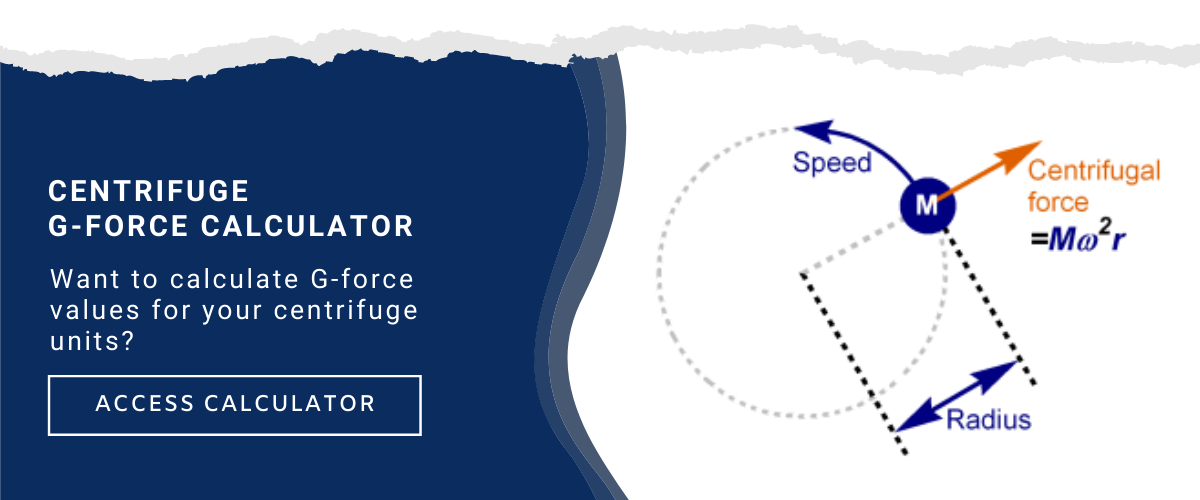When it comes to decanter centrifuges, using the right calculations to convert g-force (RCF) from revolutions per minute (RPM) or vice versa will help you achieve more precise experimental and quality control results.

Here's everything you need to know about centrifuge g-force calculations.

## What is Centrifugation?

Centrifugation is a process followed to increase the magnitude of gravitational fields so that particles in suspension experience a radial centrifugal force that moves them away from the axis of rotation.

In a decanter centrifuge, the slurry of liquid and suspended solids are fed to the centrifuge bowl and are accelerated outwards to join the pond of liquid held on the bowl's wall by the centrifugal force.

Centrifugation is used for both thickening and dewatering of sewage sludge, where dewatered sludge has a higher dry solids (DS) concentration, the centrifuge technologies used for each are almost identical.

## What is Relative Centrifugal Force?

The relative centrifugal force (RCF or the g-force) is the radial force generated by the centrifuge's spinning rotor, expressed relative to the earth's gravitational force. The g-force acting on the material's particles is exponential to the speed of rotation defined as revolutions per minute (RPM).

### What is RPM?

RPM stands for “revolutions per minute.” This is how centrifuge manufacturers communicate and display how fast materials are rotating around the axis of the rotor.

However, even at the same RPM, the centrifugal force applied to materials can vary as a function of the diameter of the rotor. So, even when spinning at the same RPM, a larger diameter will produce larger forces just as a smaller diameter will produce smaller forces.

## How to Convert RCF (G Force) to RPM and Vice Versa

Relative centrifugal force (RCF) is often used to refer to the amount of force applied when using a decanter centrifuge.

Relative centrifugal force is dependent on the speed of rotation in RPM and the distance of the particles from the center of rotation. The Relative Centrifugal Force (RCF) can be calculated when the speed of rotation is given in RPM and the distance (d) is expressed in inches.

Doubling the speed of rotation increases the centrifugal force by a factor of four. The centrifugal force also increases with the distance from the axis of rotation. These two parameters are of considerable significance when selecting the appropriate centrifuge.

#### Formulas in Inches:

RCF or G-Force = 0.00001118* ( (Bowl Diameter /2) / 0.3937)* (RPM)² )

RPM =SQRT( (G-Force / ( (Bowl Diameter /2) / 0.3937) ) / 0.00001118 )

## The Vision Machine G-Force Calculator

Use our G-Force Calculator to calculate the G-Forces exerted on samples in a centrifuge.

The calculator below will convert g-force (RCF) from revolutions per minute (RPM) or vice versa to achieve more precise experimental and quality control results that require centrifugation.#### References

G Force Calculator — RCF to RPM
https://www.sigmaaldrich.com/technical-documents/articles/biology/g-force-calculator.html

G-Force Calculator
https://druckerdiagnostics.com/g-force-calculator/